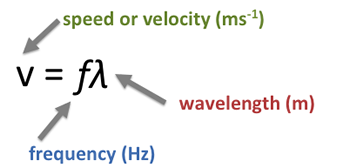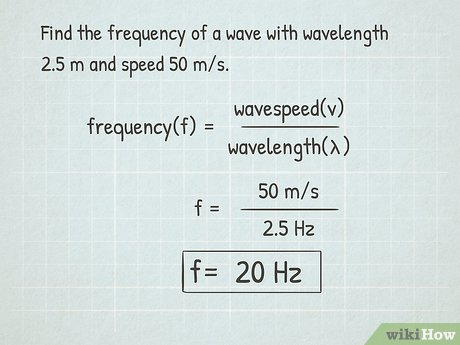Jessica

MS-PS4-1

# Wave Speed Study Guide

The distance traveled by a wave in a particular length of time is referred to as wave speed.

Table of content

## What is Speed of a Wave?

The distance a wave travels in a particular amount of time, such as the number of meters a wave travels per second, is called the velocity of the wave. Plucking a guitar string is an example of wave speed.

If someone plucks a guitar string, the strings appear to move in a transverse wave pattern. Although the sound wave is a longitudinal wave, the wave on the guitar is transverse. The particles in the string travel perpendicular to the wave's propagation direction.

## WAVE SPEED FORMULAWave speed equation (Speed = Wavelength x Frequency) relates wave speed to wavelength and frequency. When the wavelength and frequency are known, this equation can be used to calculate wave speed.

Wave frequency is the number of waves that move per second. In addition, the duration is calculated as the reciprocal of the frequency of the wave. Wave wavelength is the distance between the corresponding points of two consecutive waves.

### VARIABLES AFFECTING WAVE SPEED

• Wavelength
• Speed
• Distance
• Frequency

The above given four variables are affecting wave speed.

### THE MEDIUM'S DEPENDENCE

• The speed of most waves depends on the medium through which the wave travels.
• Waves generally pass through solids fastest and gas slowest.
• This is because the particles are closest to each other in a solid, while the particles are the closest to each other in a gas.
• If the particles are further apart, the energy distribution will take longer to pass through the medium from one particle to another.

###Properties of Waves

__1. Amplitude __

During wave motion particles oscillate about their mean position or equilibrium position to produce sound waves. The maximum displacement of the particles either above or below of the mean position is called amplitude or it can be said as Amplitude is the maximum height above or below from the mean position. Amplitude is denoted by X0 and the SI unit of amplitude is meter.

__2. Wavelength __

Transverse waves travel in consecutive or successive crest and trough while longitudinal waves travel in successive compression and rarefaction. In case of transverse waves the length of successive crust and trough is called its wavelength. While in case of longitudinal waves the length of successive compression and rarefaction is called its wavelength. Wavelength is denoted by the Greek letter lambda (λ) and its SI unit is meter.

3. Time Period

In order to understand the concept of time period we must understand the concept of wave cycle. In case of transverse waves one crust and one trough form one wave cycle or one oscillation while in case of longitudinal waves one compression and one rarefaction form one wave cycle or one oscillation. The total number of the wave cycles depend on the number of crust and trough in transverse waves whereas in the case of longitudinal waves it depends on the number of compressions and the rarefactions. The time taken by the oscillating body to complete one wave cycle or one oscillation is called time period. Time period is denoted by T and its SI unit is second. Example if a wave completes its oscillation or one wave cycle in two seconds than its time period is T = 2 seconds.

4. Frequency The number of wave cycles or number of oscillations completed in one second. Frequency is denoted by f. The SI unit of frequency is hertz. Example if a wave completes three oscillations in one second then the frequency of that wave is three hertz. Frequency is the reciprocal of the time period.

### Wave Speed Example### Summary

• Wave speed equation (Speed = Wavelength x Frequency) relates wave speed to wavelength and frequency.
• When the wavelength and frequency are known, this equation can be used to calculate wave speed.
• The frequency of a wave is the number of waves that move in one second.
• In addition, the time period is calculated as the reciprocal of the wave frequency.
• The wavelength of a wave is the distance between any two consecutive waves' corresponding points.

## FAQs

Q. What is wave speed?

The distance traveled by a given point (e.g., the summit) of a wave at a given time interval is the velocity in the case of a wave.

Q. What is the speed of a wave that has a wavelength of 2 m and a frequency of 1.5 Hz?

Speed = Wavelength x Frequency

Speed = 2 x 1.5

Speed = 3 m/sec.

Q. Sound energy travels through matter in waves. Do sound waves travel faster through air or water? Explain your answer.

Liquids are not as densely packed. Gasses, on the other hand, are relatively loosely packed. This allows sound to travel much more quickly through a solid than through a gas. In water, sound travels four times quicker and farther than it does in air.

Q. What is the formula for the speed of a wave?

Wave Speed = Wavelength x Frequency

We hope you enjoyed studying this lesson and learned something cool about Wave Speed! Join our Discord community to get any questions you may have answered and to engage with other students just like you! We promise, it makes studying much more fun!😎